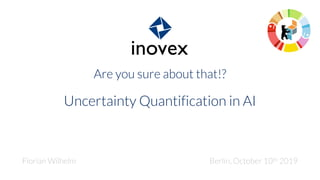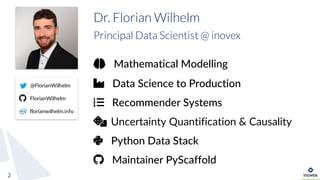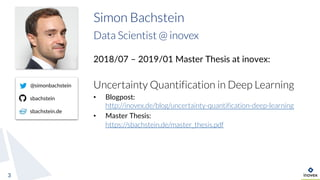Successfully reported this slideshow.

# Uncertainty Quantification in AI×

1 of 55 Ad
1 of 55 Ad

# Uncertainty Quantification in AI

With the advent of Deep Learning (DL), the field of AI made a giant leap forward and it is nowadays applied in many industrial use-cases. Especially critical systems like autonomous driving, require that DL methods not only produce a prediction but also state the certainty about the prediction in order to assess risks and failure.

In my talk, I will give an introduction to different kinds of uncertainty, i.e. epistemic and aleatoric. To have a baseline for comparison, the classical method of Gaussian Processes for regression problems is presented. I then elaborate on different DL methods for uncertainty quantification like Quantile Regression, Monte-Carlo Dropout, and Deep Ensembles. The talk is concluded with a comparison of these techniques to Gaussian Processes and the current state of the art.

With the advent of Deep Learning (DL), the field of AI made a giant leap forward and it is nowadays applied in many industrial use-cases. Especially critical systems like autonomous driving, require that DL methods not only produce a prediction but also state the certainty about the prediction in order to assess risks and failure.

In my talk, I will give an introduction to different kinds of uncertainty, i.e. epistemic and aleatoric. To have a baseline for comparison, the classical method of Gaussian Processes for regression problems is presented. I then elaborate on different DL methods for uncertainty quantification like Quantile Regression, Monte-Carlo Dropout, and Deep Ensembles. The talk is concluded with a comparison of these techniques to Gaussian Processes and the current state of the art.

### Uncertainty Quantification in AI

1. 1. Are you sure about that!? Uncertainty Quantification in AI Florian Wilhelm Berlin, October 10th 2019
2. 2. 2 Dr. Florian Wilhelm Principal Data Scientist @ inovex @FlorianWilhelm FlorianWilhelm florianwilhelm.info Mathematical Modelling Data Science to Production Recommender Systems Uncertainty Quantification & Causality Python Data Stack Maintainer PyScaffold
3. 3. 3 Simon Bachstein Data Scientist @ inovex 2018/07 – 2019/01 Master Thesis at inovex: Uncertainty Quantification in Deep Learning • Blogpost: http://inovex.de/blog/uncertainty-quantification-deep-learning • Master Thesis: https://sbachstein.de/master_thesis.pdf @simonbachstein sbachstein sbachstein.de
4. 4. 1. Motivation 2. Methods a. Gaussian Processes b. Monte-Carlo Dropout c. Deep Ensembles d. Dropout Ensembles e. Quantile Regression 3. Experiments 4. Conclusion & Outlook Agenda 4
5. 5. Deep Networks cannot look beyond their horizon Motivation 5 90% cat 10% dog
6. 6. Deep Networks cannot look beyond their horizon Motivation 6 40% cat 60% dog
7. 7. Deep Networks cannot look beyond their horizon Motivation 7 ?
8. 8. Boult, T. E., Cruz, S., Dhamija, A., Gunther, M., Henrydoss, J., & Scheirer, W. (2019). Learning and the Unknown: Surveying Steps Toward Open World Recognition. Aaai, 1–8. Retrieved from www.aaai.org Learning and the Unknown 8
9. 9. Simple Regression Problem Interpolation 9
10. 10. Simple Regression Problem Deep Networks don’t extrapolate Neural Arithmetic Logic Units, NIPS'18, Andrew Trask et. al.10
11. 11. Simple Regression Problem Deep Networks don’t extrapolate 11
12. 12. Simple Regression Problem Uncertainty about interpolation and extrapolation 12
13. 13. Types of Uncertainty 13
14. 14. 1. Motivation 2. Methods a. Gaussian Processes b. Monte-Carlo Dropout c. Deep Ensembles d. Dropout Ensembles e. Quantile Regression 3. Experiments 4. Conclusion & Outlook Agenda 14
15. 15. Methods for Uncertainty Quantification 16 Relaxation of mathematical assumptions about data Gaussian Processes Deep Ensembles / Dropout Ensembles Quantile Regression Monte Carlo Dropout
16. 16. 1. Motivation 2. Methods a. Gaussian Processes b. Monte-Carlo Dropout c. Deep Ensembles d. Dropout Ensembles e. Quantile Regression 3. Experiments 4. Conclusion & Outlook Agenda 17
17. 17. A Gaussian Process can be thought of as a random function which is defined by its mean and covariance functions Gaussian Processes 18 Definition
18. 18. Gaussian Processes 19 Example
19. 19. Gaussian Processes 20 Inference
20. 20. Gaussian Processes 21 Inference
21. 21. Gaussian Processes 22 Inference with perfect interpolation
22. 22. Gaussian Processes 23 Inference with noisy observations
23. 23. Gaussian Processes 24 Inference Inference using given data points can be done analytically. For example, when assuming the (prior) mean function to be zero everywhere, we get: Good introduction: Bayesian Non-parametric Models for Data Science using PyMC by Christopher Fonnesbeck • https://www.youtube.com/watch?v=-sIOMs4MSuA • https://de.slideshare.net/mlreview/bayesian-nonparametric-models-for-data-science-using-pymc computationally intense
24. 24. 1. Motivation 2. Methods a. Gaussian Processes b. Monte-Carlo Dropout c. Deep Ensembles d. Dropout Ensembles e. Quantile Regression 3. Experiments 4. Conclusion & Outlook Agenda 25
25. 25. MC Dropout Dropout as a Bayesian Approximation: Representing Model Uncertainty in Deep Learning, ICML 2016, Yarin Gal et. al. 26 ...
26. 26. 1. Motivation 2. Methods a. Gaussian Processes b. Monte-Carlo Dropout c. Deep Ensembles d. Dropout Ensembles e. Quantile Regression 3. Experiments 4. Conclusion & Outlook Agenda 27
27. 27. Deep Ensembles Simple and Scalable Predictive Uncertainty Estimation using Deep Ensembles, NIPS 2017, Balaji Lakshminarayanan et. al28 Custom loss function: Capture uncertainty directly at training time
28. 28. Deep Ensembles Simple and Scalable Predictive Uncertainty Estimation using Deep Ensembles, NIPS 2017, Balaji Lakshminarayanan et. al29 Combine an ensemble of networks
29. 29. 1. Motivation 2. Methods a. Gaussian Processes b. Monte-Carlo Dropout c. Deep Ensembles d. Dropout Ensembles e. Quantile Regression 3. Experiments 4. Conclusion & Outlook Agenda 30
30. 30. Dropout Ensembles 31 The best of both worlds? ...
31. 31. 1. Motivation 2. Methods a. Gaussian Processes b. Monte-Carlo Dropout c. Deep Ensembles d. Dropout Ensembles e. Quantile Regression 3. Experiments 4. Conclusion & Outlook Agenda 32
32. 32. Using the cumulative distribution function (cdf) of a random variable Y, we define the quantile: Loss function to estimate quantile: Quantile Regression 33
33. 33. Intuition behind Quantile Regression 34 0.0 0.1 0.2 0.90.3 0.4 0.5 0.6 0.7 0.8 1.0 Assume median is here (𝜏 = 0.5) 𝑦 > )𝑞+(𝑥) 𝑦 ≤ )𝑞+(𝑥) 0.1 0.2 0.5 0.8 Error: 0.0 + 1.6 = 1.6
34. 34. Intuition behind Quantile Regression 35 0.0 0.1 0.2 0.90.3 0.4 0.5 0.6 0.7 0.8 1.0 Assume median is here (𝜏 = 0.5) 𝑦 > )𝑞+(𝑥) 𝑦 ≤ )𝑞+(𝑥) 0.1 0.4 0.7 Error: 0.0 + 1.2 = 1.2 0.0
35. 35. Intuition behind Quantile Regression 36 0.0 0.1 0.2 0.90.3 0.4 0.5 0.6 0.7 0.8 1.0 Assume median is here (𝜏 = 0.5) 𝑦 > )𝑞+(𝑥) 𝑦 ≤ )𝑞+(𝑥) 0.1 0.3 0.6 Error: 0.1 + 0.9 = 1.0 0.0
36. 36. Intuition behind Quantile Regression 37 0.0 0.1 0.2 0.90.3 0.4 0.5 0.6 0.7 0.8 1.0 Assume median is here (𝜏 = 0.5) 𝑦 > )𝑞+(𝑥) 𝑦 ≤ )𝑞+(𝑥) 0.2 0.2 0.5 Error: 0.3 + 0.7 = 1.0 0.1 No change due to the linearity of the error! +0.1 +0.1 -0.1 -0.1
37. 37. Now the 0.75th Quantile 38 0.0 0.1 0.2 0.90.3 0.4 0.5 0.6 0.7 0.8 1.0 Assume 𝜏 = 0.75 is here 𝑦 > )𝑞+(𝑥) 𝑦 ≤ )𝑞+(𝑥) 0.2 0.2 0.5 0.1 Error: (1 − 0.75) ⋅ 0.3 + 0.75 ⋅ 0.7 = 0.6 Right-side error weights 3 times as much as the left-side error
38. 38. Now the 0.75th Quantile 39 0.0 0.1 0.2 0.90.3 0.4 0.5 0.6 0.7 0.8 1.0 𝜏 = 0.75 𝑦 > )𝑞+(𝑥) 𝑦 ≤ )𝑞+(𝑥) 0.5 0.1 0.2 Error: (1 − 0.75) ⋅ 1.0 + 0.75 ⋅ 0.2 = 0.4 0.4 Change in the right-side error also weights 3 times as much as the left-side error
39. 39. 1. Motivation 2. Methods a. Gaussian Processes b. Monte-Carlo Dropout c. Deep Ensembles d. Dropout Ensembles e. Quantile Regression 3. Experiments 4. Conclusion & Outlook Agenda 40
40. 40. According to the function Samples are generated as follows: Experiments Uncertainty in Deep Learning (Phd thesis), Yarin Gal, http://mlg.eng.cam.ac.uk/yarin/blog_2248.html41 Dataset
41. 41. Experiments 42 Dataset
42. 42. Neural networks › 2 hidden layers with 20 ReLU neurons each › 5 networks for Deep Ensembles › 100 iterations for Dropout predictions › Adam optimizer with batch size of 128 › LR, weight decay, dropout probability are optimized Gaussian Processes › squared exponential covariance and zero mean function prior › covariance function parameters and aleatory noise are optimized Experiments 43 Network setup and hyperparameters
43. 43. Mean squared error (MSE) Mean negative log likelihood (MNLL) Mean Kullback-Leibler (KL) divergence Experiments 44 Measures for generalization quality
44. 44. Experiments 45 Interpolation
45. 45. Experiments 46 They still don’t extrapolate and they don’t quite realize
46. 46. Experiments 47 Gaussian Process
47. 47. Experiments Convergence 48
48. 48. Experiments 49 Heteroscedastic noise
49. 49. Experiments 50 Non-Gaussian noise
50. 50. Experiments 51 Uncertainty split aleatoric epistemic
51. 51. Summary 52 GP MCD DeepE DropoutE QR Homoscedastic noise ++ o + o o Heteroscedastic noise -- - ++ + + Non-Gaussian noise + o + + - Convergence ++ - + - + Speed (--) + - / (+) + ++ Uncertainty split yes no yes yes no
52. 52. 1. Motivation 2. Methods a. Gaussian Processes b. Monte-Carlo Dropout c. Deep Ensembles d. Dropout Ensembles e. Quantile Regression 3. Experiments 4. Conclusion & Outlook Agenda 53
53. 53. › Neural network approaches discussed here are very aware of aleatory uncertainty, however, not capable of correctly estimating epistemic uncertainty › Gaussian Processes give clear signals about ignorance but do not scale A combined solution needs to be developed because uncertainty estimation is needed in critical applications Conclusion 54 There is work to be done
54. 54. › Bayesian Neural Networks (e.g. with PyMC) › Sparse Gaussian Process approximations › Gaussian Processes on top of neural networks Outlook 55 Other approaches
55. 55. Thank You! Florian Wilhelm Principal Data Scientist inovex GmbH Schanzenstraße 6-20 Kupferhütte 1.13 51063 Köln florian.wilhelm@inovex.de